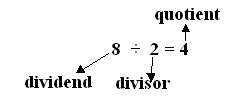Email us to get an instant 20% discount on highly effective K-12 Math & English kwizNET Programs!

#### Online Quiz (WorksheetABCD)

Questions Per Quiz = 2 4 6 8 10

### MEAP Preparation - Grade 6 Mathematics2.45 Division Vocabulary

 Explanation: Addition, subtraction, multiplication and division are called operations. Subtraction is the inverse of addition. Multiplication is the inverse of division. Multiplication is repeated addition. Division is repeated subtraction. Zero divided by any number (except 0 equals 0) Number divided by any 0 is not defined Any number(except 0) divided by itself equals 1. Any number divided by 1 equals that number. The answer to the division problem is called the quotient. The number you are dividing is called the dividend. The number you are dividing by is called the divisor.Example: What times 5 equals 20? From 5 tables, we have 5 * 4 = 20 Answer: 4 Directions: Answer the following questions. Explain the division vocabulary in your own words. Also, write the times tables from one through ten.
 Q 1: For 6 x 7 = 42, the corresponding division fact is6/7 = 426/7 = 4242/7 = 6 Question 2: This question is available to subscribers only!